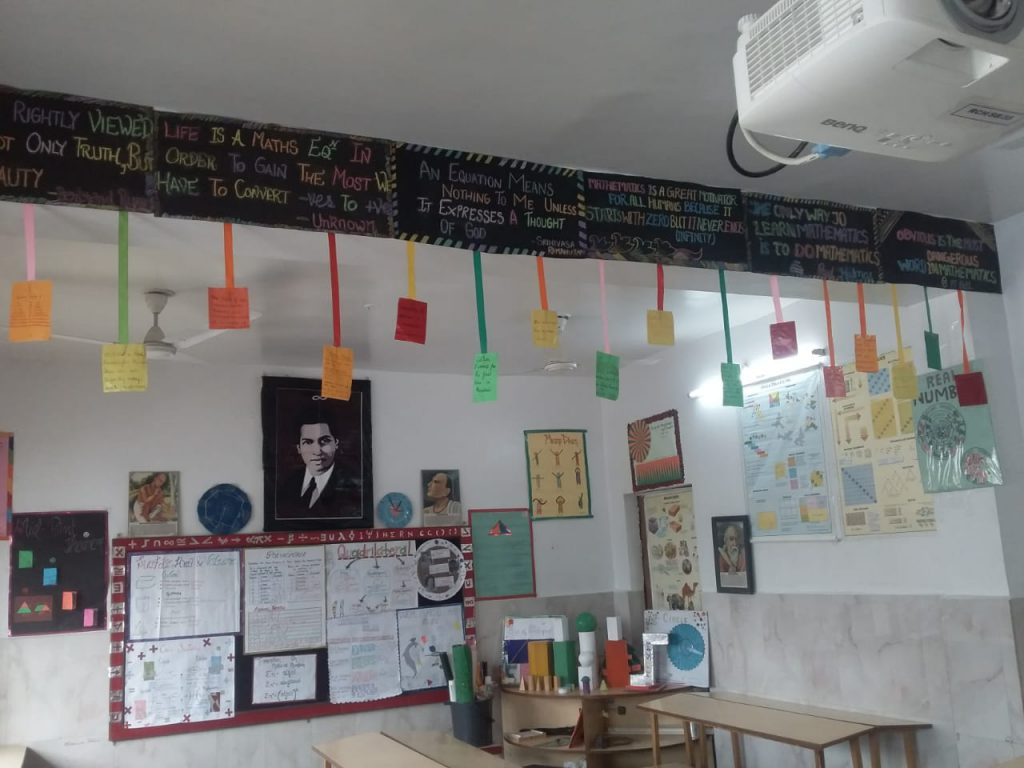Mathematics Room

## Mathematics RoomMathematics Lab

The school has well equipped Maths Lab to make the subject more enjoyable and practical for the students. The laboratory is aptly named Ramanujam Mathematics Laboratory It embodies the spirit of exploration and teaches the child to observe keenly, reflect on ideas logically, think independently, innovatively and analyze intricately . Number of activities are conducted with colorful paper cutouts & paper folding activities which help to clarify simple concepts of Mathematics.

The geometric sketch pad and the handheld calculators are used to explain concepts from coordinate geometry to Calculus, to learn and understand quadratic equations; conic sections and trigonometric curves etc. Interactive mental maths quizzes and speed calculation competitions are also held in the lab to make mathematics learning interesting.